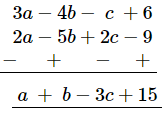# Subtract: 2a − 5b + 2c − 9 from 3a − 4b − c + 6

Question:

Subtract:
2a − 5b + 2c − 9 from 3a − 4b − c + 6

Solution:

Writing the terms of the given expressions (in the same order) in the form of rows with like terms below each other and subtracting column-wise: